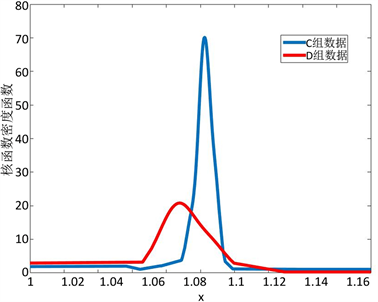# 迁移学习在超声波流量计数据分析及故障诊断上的应用Application of Transfer Learning in Data Analysis and Fault Diagnosis of Ultrasonic Flowmeter

DOI: 10.12677/AAM.2019.82021, PDF, HTML, XML, 下载: 554  浏览: 936

Abstract: As an important industrial measuring equipment, ultrasonic flowmeter is widely used in fluid de-tection. Flowmeters with different designs collect different characteristics and generate a large amount of data at every time, every minute and every place. These data have different similarities and structures. Traditional machine learning cannot efficiently use such data. This kind of problem requires transfer learning method. In this paper, we deal with similar data generated by different flowmeters, transfer learning under the condition of higher data similarity, and obtain better results.

1. 引言

2. 算法介绍

1、初始权重向量 ${w}^{1}=\left({w}_{1}^{1},\cdots ,{w}_{n+m}^{1}\right)$ ，其中，

${w}_{i}^{1}=\left\{\begin{array}{l}\frac{1}{n}\text{\hspace{0.17em}}\text{\hspace{0.17em}}\text{\hspace{0.17em}}\text{\hspace{0.17em}}i=1,\cdots ,n\\ \frac{1}{m}\text{\hspace{0.17em}}\text{\hspace{0.17em}}\text{\hspace{0.17em}}i=n+2,\cdots ,n+m\end{array}$

2、初始 $\beta$

$\beta =\frac{1}{1+\sqrt{2\mathrm{ln}\left(nN\right)}}$

For $t=1,\cdots ,N$

1、归一化权重 ${p}^{t}=\frac{{w}^{t}}{{\sum }_{i=1}^{n+m}{w}_{i}^{t}}$

2、利用带权数据计算得到分类器 ${P}_{t}$ ，计算该分类器在 ${T}_{t}$ 上的错误率 ${c}_{t}$

3、 $\beta =\frac{{c}_{t}}{1-{c}_{t}}$

${w}_{i}^{t+1}=\left\{\begin{array}{l}{w}_{i}^{t}{\beta }^{|{P}_{t}\left({x}_{i}\right)-c\left({x}_{i}\right)|}\text{\hspace{0.17em}}\text{\hspace{0.17em}}\text{\hspace{0.17em}}\text{\hspace{0.17em}}i=1,\cdots ,n\\ {w}_{i}^{t}{\beta }_{t}^{-|{P}_{t}\left({x}_{i}\right)-c\left({x}_{i}\right)|}\text{\hspace{0.17em}}\text{\hspace{0.17em}}\text{\hspace{0.17em}}i=n+2,\cdots ,n+m\end{array}$

3. 实例研究

3.1. 数据来源

3.2. 实验结果

4. 结论Figure 1. Kernel density function curveTable 4. Traditional machine learning results

  陈鹏, 任霖钦, 蒋安荔. 超声波流量计诊断及故障处理[J]. 石化技术, 2016, 23(3): 133.  庄福振, 罗平, 何清, 史忠植. 迁移学习研究进展[J]. 软件学报, 2015, 26(1): 26-39.  戴文渊. 基于实例和特征的迁移学习算法研究[D]: [硕士学位论文]. 上海: 上海交通大学, 2009.  张倩, 李海港. 基于知识表达的迁移学习方法及其应用[M]. 徐州: 中国矿业大学出版社, 2015: 36-39.  Ultrasonic Flowmeter Diagnostics Data Set. http://archive.ics.uci.edu/ml/datasets/Ultrasonic+flowmeter+diagnostics#